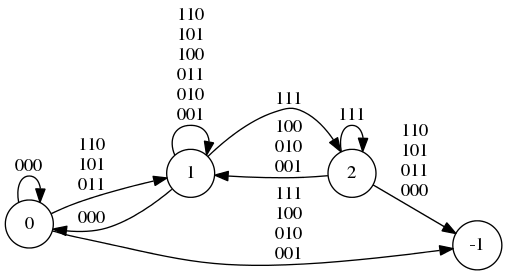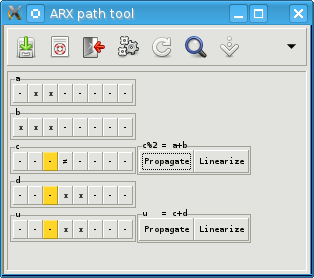# ARX Toolkit

## Description

The ARX toolkit is a set of tools to study ARX ciphers and hash functions. This is still a work in progress, and this website will be updated soon with more content. The latest version of the toolkit available from this page corresponds to the Crypto 2013 paper; however some documentation is still missing, and an updated version should be available soon.

(Last update: small update to the documentation and code fixes in April 2015)

The ARX toolkit has been described in two papers:

## Part I: Study S-functions using Finite State Machines

The first part is a tool to build Finite State Machines or Automata from a simple description of a system of Addition, Xor, and Boolean function. The input is system is described with a natural syntax, and the output automaton uses the following conventions:

• The parameters are read from the LSB to the MSB.
• The initial state is state 0
• The dead state is state -1
• All other states are accepting states

### Syntax

SyntaxDescription
Vi Variable number i.
Pi Parameter number i.
Mi: 〈…〉; Macro definition.
Xi Macro parameter inside a macro definition.
Mi(〈…〉, …) Macro call. Parameters are separated by commas.
Muxi Multiplexer (2i-to-1). This expect i control inputs, and 2i data inputs. Warning: do not put any addition inside the data inputs this will give incorrect results.
+-^|& Addition, substraction, xor, or, and. Priority order is left to rigth.
==^^||&& Boolean operations. These are equivalent to single characters version, excepted that they have higher priority.
() Use parenthesis for grouping. The is better than relying of operations priority.
01 Constants.
; Equations must be delimited by semi-colons.
# Comment. Ignore everything until end of line.

### Options

OptionDescription
-t Output transition FSM
-d Output decision FSM
-o 〈file〉 Output file
-e Take expression from command line
-i Read from standard input
-v Verbose (debug output)
-b Dump in binary format instead of C format
-g Output in Graphviz format

### Example 1: compatible XOR differences for additionWe can use this tool to find out which xor-differences are possible for an addition. For a given dx,dy,dz we want to know whether the exists x and y such that (x⊕dy)+(y⊕dy)=(x+y)⊕dz. We can use this tool to build an automaton that will accept exactly those dx,dy,dz.

The automaton on the right was build with the following command: ```build_fsm -e '(V0^P0)+(V1^P1)==(V0+V1)^P2' -v -d -g -dead | dot -Tpdf```. From this automaton it is easy to see that the differences that are not compatible are exactly the differences satisfying one of those conditions:

• dx⊕dy⊕dz=1
• ∃ i: dx[i]=dy[i]=dz[i]=0 and dx[i+1]⊕dy[i+1]⊕dz[i+1]=1
• ∃ i: dx[i]=dy[i]=dz[i]=1 and dx[i+1]⊕dy[i+1]⊕dz[i+1]=0

### Example 2: Differential properties of the additions using our constraints set

We can build the automaton needed for the second part with `build_fsm add.system -d -b -v -o add.dfsm`, and `build_fsm add.system -t -b -v -o add.tfsm`.

## Part II: Differential properties of ARX operations

The second part is a tool to study differential properties of ARX operations. We use an approach similar to the Generalized characteristics of de Cannière and Rechberger (Asiacrypt 2006), but we introduce a new constraint set (see the paper for details).

Important note: this section describes the tools corresponding to the Asiacrypt 2012 paper, but an improved set of constraints has been proposed at Crypto 2013. See Part VI for a quick description of how to use the tools with the new constraints.

This tool requires the operation to be represented by an automaton, as computed with first tool, and can be used for any operation that can be written as an S-system. It can compute the probability of a path to be followed, and propagate constraints (i.e. it find necessary conditions).

### Example 1: compute xor-probabilities through an addition

We can compute differential probabilities of the addition. For instance, let us consider a 4-bit addition, with the LSB of both inputs active, and the output inactive:

• a+b = c
• a'+b' = c'
• a' = a ⊕ 1
• b' = b ⊕ 1
• c' = c

We run the tool as follows:

```\$ constraints -d add.dfsm -t add.tfsm -w 4 -c -n 4 -- "---x" "---x" "----"
System is compatible!
#Sol: 128 (2^7.0)
Cost: 1.0
Cost: 1.0
Cost: 1.0
```

The tools computes that there are 128 solutions for (a,b,c). Moreover, the probability that:

• a satisfies the system, for a random choice of valid b and c is 2-1
• b satisfies the system, for a random choice of valid a and c is 2-1
• c satisfies the system, for a random choice of valid a and b is 2-1

The last item can be written as xdp+(0x1,0x1->0x0)=1/2.

### Example 2: propagate constraints

Let us consider the same operation and propagate constraints:

```\$ constraints -d add.dfsm -t add.tfsm -w 4 -p -n 4 -- "---x" "---x" "----"
System is compatible!
Propagate:
Sign constraint: 2.0:1
1 new constraints
New parameters: ---x ---x ---1
```

The tool detect one new constraint: the LSB of the output must be 1.

### Example 3: compute probabilities through an addition

We can also use extended constraints and compute the probability of more complex differential paths. For instance let us use the constraints of the previous example:

```\$ constraints -d add.dfsm -t add.tfsm -w 4 -c -n 4 -- "---x" "---x" "---1"
System is compatible!
#Sol: 128 (2^7.0)
Cost: -0.0
Cost: -0.0
Cost: 1.0
```

The tools computes that there are still 128 solutions for (a,b,c). This is expected, since the new constraint is a necessary constraint.

Moreover, the probability that:

• a satisfies the system, for a random choice of valid b and c is 1
• b satisfies the system, for a random choice of valid a and c is 1
• c satisfies the system, for a random choice of valid a and b is 2-1

## Part III: Analysis of differential pathsThe third part is a tool to study differential paths in ARX constructions. A simple text is used to describe the cipher and the differential path, and the tool can be used to modify the path by hand, and to compute necessary conditions (sometimes, this allows to detect contradictions).

The tool can be used to study a single differential path, or a set of four paths used in a boomerang attack

The path is displayed as a series of registers corresponding to each intermediate value in the cipher, and each register is seen as a collection of individual bits. The individual bits are represented using our new set of constraints, and the constraints can be modified by hang by clicking them:

• left click cycles through ?, - and x
• right click allow to access more constrained states: 0 and 1 from -; and u and n from x

In case of inconsistencies, the bits that are involved are highlighted. You can use this to manually modify the path.

The buttons in the toolbar are the following:

• Save
• Help
• Quit
• Propagate: propagate constraints for all equations
• Linearize: propagate constraints assuming that additions behave like xors.
• Toggle hidden states: some temporary registers can be shown or hidden (this feature does not work very well at this point: it take a very long time to refresh the display)
• Push state: push the current state in a stack
• Pop state: pop the last state from the stack. This can be used as a form of undo.

Examples of differential paths are included to show the syntax of the path file.

Important note: large paths take a long time to load (several minutes).

## Part IV: Automatic construction of differential characteristics

At Crypto 2013, we described an algorithm to build differential characteristics automatically for Skein-256. The corresponding code is available from the download section below.

These results are based on a new set of constraints. An example of system with the constraints is given below. The automata should now be built with ```build_fsm add-xx.system -s -o add-xx.fsm```, and used with ```constraints_xx -s add-xx.fsm -w 4 -p -- "---x" "---x" "----"```. The tools available for download use these new automatons.

More detailed explanations to use the code will be available later...